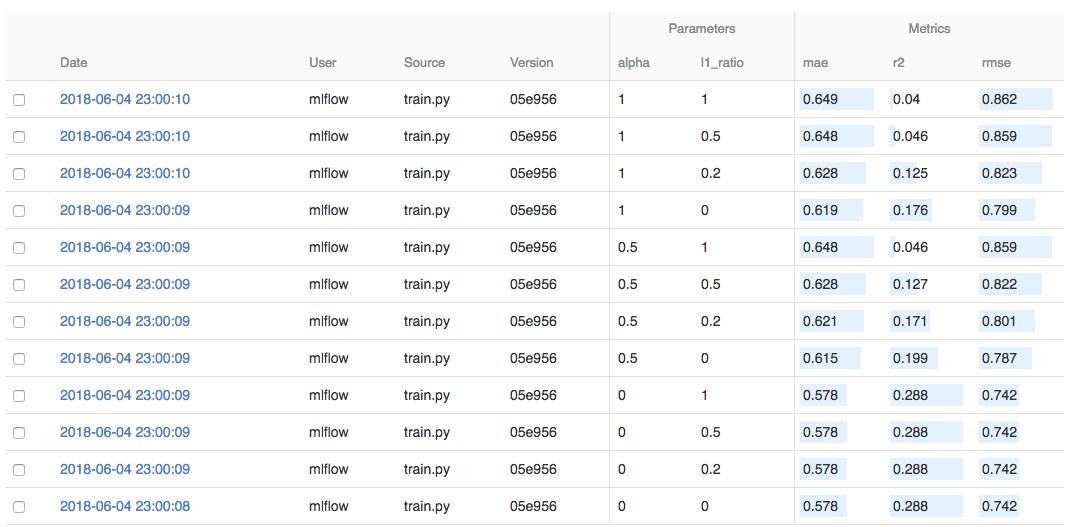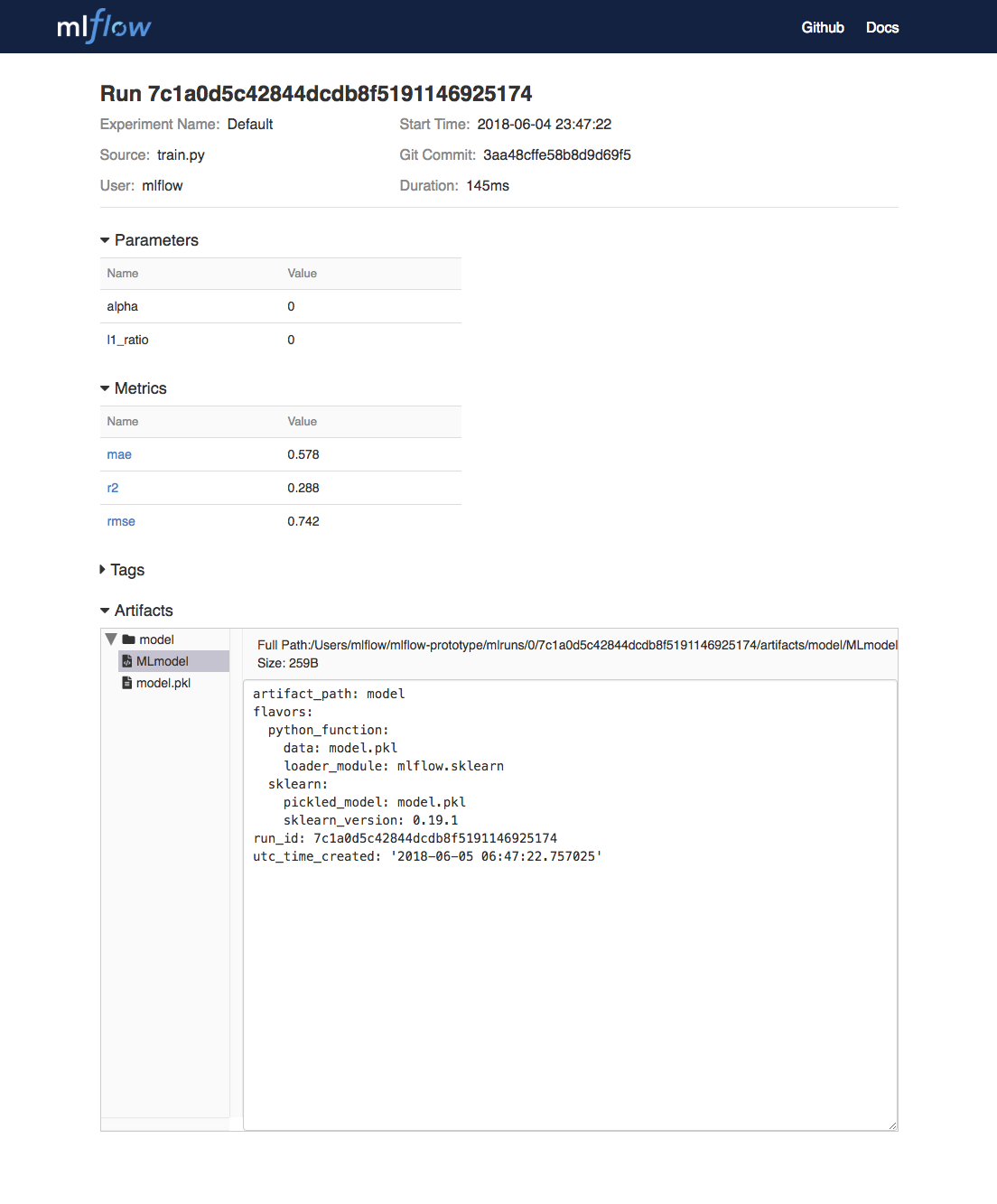2018/06/07 09:42

# Spark机器学习工具链-MLflow使用教程

## 你首先需要？

git clone https://github.com/databricks/mlflow

## 训练模型

# Read the wine-quality csv file (make sure you're running this from the root of MLflow!)
wine_path = os.path.join(os.path.dirname(os.path.abspath(__file__)), "wine-quality.csv")

# Split the data into training and test sets. (0.75, 0.25) split.
train, test = train_test_split(data)

# The predicted column is "quality" which is a scalar from [3, 9]
train_x = train.drop(["quality"], axis=1)
test_x = test.drop(["quality"], axis=1)
train_y = train[["quality"]]
test_y = test[["quality"]]

alpha = float(sys.argv) if len(sys.argv) > 1 else 0.5
l1_ratio = float(sys.argv) if len(sys.argv) > 2 else 0.5

with mlflow.start_run():
lr = ElasticNet(alpha=alpha, l1_ratio=l1_ratio, random_state=42)
lr.fit(train_x, train_y)

predicted_qualities = lr.predict(test_x)

(rmse, mae, r2) = eval_metrics(test_y, predicted_qualities)

print("Elasticnet model (alpha=%f, l1_ratio=%f):" % (alpha, l1_ratio))
print("  RMSE: %s" % rmse)
print("  MAE: %s" % mae)
print("  R2: %s" % r2)

mlflow.log_param("alpha", alpha)
mlflow.log_param("l1_ratio", l1_ratio)
mlflow.log_metric("rmse", rmse)
mlflow.log_metric("r2", r2)
mlflow.log_metric("mae", mae)

mlflow.sklearn.log_model(lr, "model")

python example/tutorial/train.py

python example/tutorial/train.py <alpha> <l1_ratio>

## 比较模型## 打包训练代码

# example/tutorial/MLproject

name: tutorial

conda_env: conda.yaml

entry_points:
main:
parameters:
alpha: float
l1_ratio: {type: float, default: 0.1}
command: "python train.py {alpha} {l1_ratio}"
# example/tutorial/conda.yaml

name: tutorial
channels:
- defaults
dependencies:
- numpy=1.14.3
- pandas=0.22.0
- scikit-learn=0.19.1
- pip:
- mlflow

Projects can also be run directly from Github if the repository has a MLproject file in the root. We’ve duplicated this tutorial to the https://github.com/databricks/mlflow-example repository which can be run with mlflow run git@github.com:databricks/mlflow-example.git -P alpha=0.42.

## 服务模型

mlflow.sklearn.log_model(lr, "model")mlflow sklearn serve /Users/mlflow/mlflow-prototype/mlruns/0/7c1a0d5c42844dcdb8f5191146925174/artifacts/model -p 1234

curl -X POST -H "Content-Type:application/json" --data '[{"fixed acidity": 6.2, "volatile acidity": 0.66, "citric acid": 0.48, "residual sugar": 1.2, "chlorides": 0.029, "free sulfur dioxide": 29, "total sulfur dioxide": 75, "density": 0.98, "pH": 3.33, "sulphates": 0.39, "alcohol": 12.8}]' http://127.0.0.1:1234/invocations

# RESPONSE
# {"predictions": [6.379428821398614]}

## 更多资源

0
1 收藏

### 作者的其它热门文章0 评论
1 收藏
0